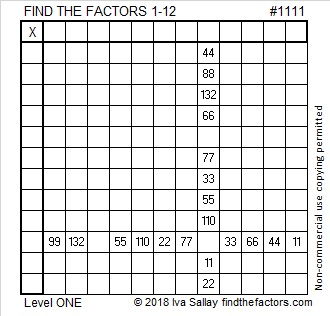# 1111 and Level 1

This is puzzle number 1111, a number made with four 1’s. The puzzle number doesn’t usually have anything to do with the puzzle, but I made an exception this time:  One of the factors of 1111 is important in solving this particular level 1 puzzle. Have fun solving it!Print the puzzles or type the solution in this excel file: 12 factors 1111-1119

Here are a few things I’ve learned about the number 1111:

• 1111 is a composite number.
• Prime factorization: 1111 = 11 × 101
• The exponents in the prime factorization are 1 and 1. Adding one to each and multiplying we get (1 + 1)(1 + 1) = 2 × 2 = 4. Therefore 1111 has exactly 4 factors.
• Factors of 1111: 1, 11, 101, 1111
• Factor pairs: 1111 = 1 × 1111 or 11 × 101
• 1111 has no square factors that allow its square root to be simplified. √111133.33166661111 is the hypotenuse of exactly one Pythagorean triple:
220-1089-1111 which is 11 times (20-99-101)

1111² = 1234321, a very special palindrome!

1111 is a repdigit in base 10, and it is a palindrome in three consecutive bases plus one more:
It’s 787 in BASE 12 because 7(144) + 8(12) + 7(1) = 1111,
676 in BASE 13 because 6(169) + 7(13) + 6(1) = 1111,
595 in BASE 14 because 5(196) + 9(14) + 5(1) = 1111, and it’s
171 in BASE 30 because 1(900) + 7(30) + 1(1) = 1111

This site uses Akismet to reduce spam. Learn how your comment data is processed.